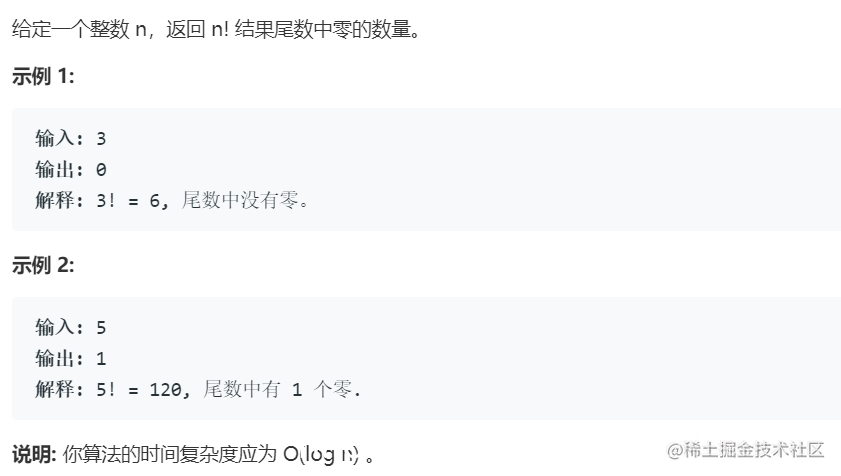# 计算阶乘之后的零个数

## 问题描述## 问题解决

``````11! = 11 * 10 * 9 * 8 * 7 * 6 * 5 * 4 * 3 * 2 * 1
=
11 * (2 * 5) * 9 * (4 * 2) * 7 * (3 * 2) * (1 * 5) * (2 * 2) * 3 * (1 * 2) * 1
``````

``````public int trailingZeroes(int n) {
int count = 0;
for (int i = 1; i <= n; i++) {
int N = i;
while (N > 0) {
if (N % 5 == 0) {
count++;
N /= 5;
} else {
break;
}
}
}
return count;
}
``````

``````public int trailingZeroes(int n) {
int count = 0;
while (n > 0) {
count += n / 5;
n = n / 5;
}
return count;
}
``````